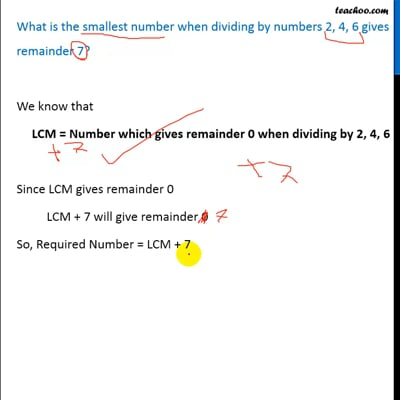Statement questions on LCM

Chapter 3 Class 6 Playing with Numbers
Concept wise

We know that

LCM = Number which gives remainder 0 when dividing by 2, 4, 6

Since LCM gives remainder 0

LCM + 7 will give remainder 0

So, Required Number = LCM + 7

LCM of 2, 4, 6 = 12

Required Number = LCM + 7

= 12 + 7

= 19

Same concept is used in Example 14 and Ex 3.7.4This video is only available for Teachoo black users

Get live Maths 1-on-1 Classs - Class 6 to 12